# MA.912.NSO.1.6Export Print
Given a numerical logarithmic expression, evaluate and generate equivalent numerical expressions using the properties of logarithms or exponents.

### Clarifications

Clarification 1: Within the Mathematics for Data and Financial Literacy Honors course, problem types focus on money and business.
General Information
Subject Area: Mathematics (B.E.S.T.)
Strand: Number Sense and Operations
Status: State Board Approved

## Benchmark Instructional Guide

### Terms from the K-12 Glossary

• Base
• Exponent
• Expression
• Inverse function

### Vertical Alignment

Previous Benchmarks

Next Benchmarks

### Purpose and Instructional Strategies

In grade 8, students generated equivalent numerical expressions and evaluated expressions using the Laws of Exponents with integer exponents. In Algebra I, students worked with rational-number exponents. In Mathematics for College Algebra, students extend the Laws of Exponents to the properties of logarithms.
• Instruction makes the connection between the properties of logarithms and the properties of exponents. Explain that a logarithm is defined as an exponent establishing the equivalence of $y$ = $a$$x$ and, $x$ = log$a$ given $a$ > 0 and $a$ ≠ 1 (MTR.5.1). Remind students that logarithmic and exponential operations are inverse operations, so the properties of the logarithms are the “opposite” of the properties of the exponents.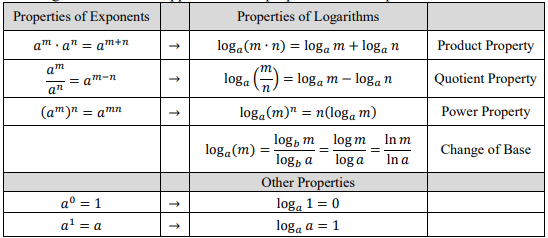• Instruction includes making the connection between the change of base formula and the inverse relationship between exponents and logarithms.
• For example, students should know that by definition $b$log$b$$x$ = $x$. Therefore, students can take the log with base $a$ of both sides of the equation to obtain log$a$ $b$log$b$$x$ = log$a$$x$. Then, students can use the the Power Property to rewrite the equation as (log$b$$x$)(log$a$$b$) = log$a$$x$. Students should notice that there the arguments for two of the logs are $b$ and $x$ with each the same base of $a$. So, one can divide both sides of the equation by log$a$ $b$ to isolate log$b$ $x$, obtaining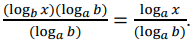Therefore,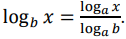• Instruction encourages students to read the logarithmic expressions and then discuss the meaning before trying to evaluate them or use the properties (MTR.4.1)
• For example, present students with log1.03 1.092727 and ask them for its meaning, “the exponent required on the base 1.03 to obtain 1.092727.”
• Many examples in this course will require students to utilize the change of base formula to evaluate logarithms. Consider the example above.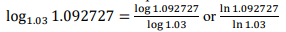• Evaluating this expression with a calculator requires the use of common logs or natural logs. Guide students to understand that common logs have a base of 10 while natural logs have a base of $e$ and are easy to enter into a calculator. Have students use a calculator to evaluate the expression to find it is approximately equivalent to 3. Have students confirm this by checking the exponential equivalent expression 1.033= 1.092727.
• While students will see more complex logarithms in this course, it is appropriate to simplify the logarithms they work with initially in this benchmark until they reach an understanding of the properties of logarithms. Depending on the courses students have taken prior to Math for College Algebra, this may their first introduction to logarithms.
• Instruction includes the understanding that logarithms with base 10 are called common logarithms and written as log $x$ or log10 $x$
• Instruction includes the understanding that logarithms with base $e$ are called natural logarithms and written as ln $x$ or log$e$ $x$
• Instruction encourages students to read the logarithmic expressions and then discuss the meaning before trying to evaluate it or use the properties.
• For example, present students with log2 64 and ask for its meaning, “the exponent required on the base 2 to obtain 64” (MTR.2.1)
• Students should understand how to use their one-to-one and inverse properties to evaluate expressions by making decisions on which base is best to use for solving.
• One-to-One Properties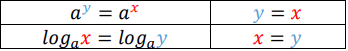• Inverse Properties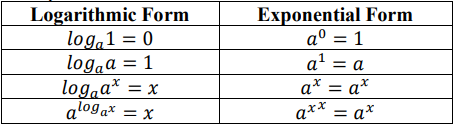• Students should have practice combining the properties of logarithms to generate equivalent algebraic expressions by either simplifying (condensing) or expanding the expression.
• Problem types include logarithms with different bases, including common logarithms and natural logarithms.

### Common Misconceptions or Errors

• Students may see “log” as a variable rather than as an operation.
• For example, they may see “log” as a common factor in the expression, log 12 + log 6 and mistakenly write log(12 + 6).
• For example, they may rewrite the expression log(12 · 6) and as log 12 · log 6 instead of log 12 + log 6. Similarly, they may incorrectly rewrite log ($\frac{\text{12}}{\text{6}}$) as $\frac{\text{log 12}}{\text{log 6}}$ instead of log 12 − log 6.
• For example, they may cancel the “log” from the numerator and the denominator in an expression.
• Students may cancel the “log” from the numerator and the denominator in an expression. Remind students that just like with roots we can simplify or expand logarithms if the argument is fully factored.

• Recall that log$b$($x$) is by definition the exponent which $b$ must be raised to in order to yield $x$ ($b$ > 0).
• Part A.
• Use this definition to compute log2(25).
• Use this definition to compute log10(0.001).
• Use this definition to compute ln($e$3).
• Explain why log$b$ ($b$$y$) = $y$ where $b$ > 0.
• Part B.
• Evaluate 10logln(100).
• Evaluate 2log2(√2)
• Evaluate $e$ln(89)
• Explain why $b$log$b$($x$) = $x$ where $b$ > 0.

### Instructional Items

Instructional Item 1 (MTR.3.1
• Use the properties of logarithms to rewrite each expression into lowest terms.
a. log2 25
b. log3 $\frac{\text{12}}{\text{5}}$
c. log5 3 + log5 13

Instructional Item 2
• What is the value of the logarithmic expression log2(26)

Instructional Item 3

• Which of the following expressions are equivalent to log416? Select all that apply.
a. $\frac{\text{log 4}}{\text{log 16}}$

b. $\frac{\text{log 16}}{\text{ln 4}}$

c. $\frac{\text{ln 4}}{\text{ln 16}}$

d. $\frac{\text{ln 16}}{\text{ln 4}}$

e. $\frac{\text{ln 4}}{\text{log 6}}$

f. $\frac{\text{log 16}}{\text{log 4}}$

*The strategies, tasks and items included in the B1G-M are examples and should not be considered comprehensive.

## Related Courses

This benchmark is part of these courses.
1200330: Algebra 2 (Specifically in versions: 2014 - 2015, 2015 - 2022, 2022 and beyond (current))
1200340: Algebra 2 Honors (Specifically in versions: 2014 - 2015, 2015 - 2022, 2022 and beyond (current))
1200400: Foundational Skills in Mathematics 9-12 (Specifically in versions: 2014 - 2015, 2015 - 2022, 2022 and beyond (current))
7912095: Access Algebra 2 (Specifically in versions: 2016 - 2018, 2018 - 2019, 2019 - 2022, 2022 and beyond (current))
1200388: Mathematics for Data and Financial Literacy Honors (Specifically in versions: 2022 and beyond (current))
1200710: Mathematics for College Algebra (Specifically in versions: 2022 and beyond (current))

## Related Access Points

Alternate version of this benchmark for students with significant cognitive disabilities.
MA.912.NSO.1.AP.6: Given a numerical logarithmic expression, identify an equivalent numerical expression using the properties of logarithms or exponents.

## Related Resources

Vetted resources educators can use to teach the concepts and skills in this benchmark.

## Student Resources

Vetted resources students can use to learn the concepts and skills in this benchmark.

## Parent Resources

Vetted resources caregivers can use to help students learn the concepts and skills in this benchmark.